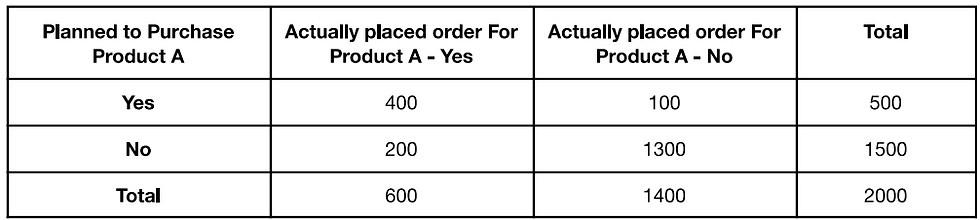top of page
Search

# Applied Statistics Assignment Help | Sample Question Paper

Part 1

Use below table to answer the given below questions.Q 1. A Use above table to find the joint probability of the people who planned to purchase and actually placed and order.

Q 1. B Use above table to find the joint probability of the people who planned to purchase and actually placed and order, given that people planned to purchase.

Q 2. An electrical manufacturing company conducts quality checks at specified periods on the products it manufactures. Historically, the failure rate for the manufacturing item is 5%. Suppose a random sample of 10 manufactured items is selected. Answer the following questions.

A. Probability that none of the items are defective?

B. Probability that exactly one of the items is defective?

C. Probability that two or fewer of the items are defective?

D. Probability that three or more of the items are defective?

Q3. A car salesman sells on an average 3 cars per week.

A. What is probability that in a given week he will sell some cars.

B. What is probability that in a given week he will sell 2 or more but less that 5 cars.

C. Plot the Poisson distribution function for cumulative probability of cars sold per-week ns number of cars sold per week.

Q4. Accuracy in understanding orders for a speech based bot at a restaurant is important for the Company X which has designed, marketed and launched the product for a contactless delivery due to the COVID-19 pandemic. Recognition accuracy that measures the percentage of orders that are taken correctly is 86.8%. Suppose that you place an order with the bot and two friends of your independently place orders with the same bot. Answer the following questions.

A. What is the probability that all three orders will be recognised correctly.

B. What is the probability that none of the three orders will be recognised correctly.

C. What is the probability that at least two of the three orders will be recognised correctly?

Q5. Explain 1 real life industry scenario(other than the ones mentioned above) where you can use the concepts learnt in this module of Applied statistics to get a data driven business solution.

Code Implementation

```# importing libraries
import pandas as pd
import numpy as np
import warnings
import matplotlib.animation as animation
import seaborn as sns
import math
import matplotlib.pyplot as plt

sns.set(style="darkgrid")
pd.set_option('display.max_columns', 100)
pd.set_option('display.max_colwidth', -1) # data and columns are displayed correctly
pd.options.display.float_format = '{:20,.2f}'.format # display float value with correct precision

warnings.filterwarnings('ignore')```

## Que1a

```##You observe from the above table, that 400 people planned to purchase and actually placed an order  is
##400 out of 2000 people.

plan_purchase = 400
total = 2000
joint_prob = round(plan_purchase/total,4)
joint_prob```

Output:

0.2

```# P1 = P(Actually placed an order | Planned to purchase)
# P2 = Planned to purchase and actually placed an order
# P3 = Planned to purchase
## P1 = P2/P3
P1   = (400 / 2000 )/ (500 / 2000)```

## Que2

```import numpy as np
import pandas  as pd
import scipy.stats as stats
import matplotlib.pyplot as plt
p   =  0.05 # failure rate of products that the company manufactures is 5%
n   =  10 # sample size
k   =  np.arange(0,11) # An array of quantiles

binomial = stats.binom.pmf(k,n,p)
print(binomial)```

Output:

[5.98736939e-01 3.15124705e-01 7.46347985e-02 1.04750594e-02 9.64808106e-04 6.09352488e-05 2.67259863e-06 8.03789063e-08 1.58642578e-09 1.85546875e-11 9.76562500e-14]

## que2a

`binomial`

Output:

0.5987369392383789

## que2b

`binomial`

Output:

0.31512470486230504

## que2c

```cumbinomial = stats.binom.cdf(k,n,p)
cumbinomial```

Output:

0.9884964426207031

## que2d

```P = 1- cumbinomial
P```

Output:

0.01150355737929687

## Que3

```rate =  3 # which is the mean
n    =  np.arange(0,16)
cumpoisson = stats.poisson.cdf(n,rate)
cumpoisson```

Output:

array([0.04978707, 0.19914827, 0.42319008, 0.64723189, 0.81526324, 0.91608206, 0.96649146, 0.9880955 , 0.99619701, 0.99889751, 0.99970766, 0.99992861, 0.99998385, 0.9999966 , 0.99999933, 0.99999988])

## que3a

```P   = 1 - cumpoisson
P```

Output:

0.950212931632136

## que3b

```# Let P1 be the probability that the salesman sells more than 4 cars
P1 = cumpoisson # P(X >=4)
# Let P2 be the probability that the salesman sells more than 1 cars
P2 = cumpoisson # P(X >=1)
P = P1 - P2 # Prob. that the salesman will sell between 2 and 5 cars
P```

Output:

0.6161149710523164

## que3c

```poisson = stats.poisson.pmf(n,rate)
plt.plot(n,poisson, 'o-')
plt.title('Poisson')
plt.xlabel('Number of cars sold per week')
plt.ylabel('Cumulative Prob of cars sold per week')
plt.show()```

Output:## Que4

```#Because there are three orders and the probability of a correct order is 0.868.
#    Using Binomial distribution equation,

P(X = 3|n =3, pi given = 0.868)
3!/ 3!(3-3)! * (0.868)^3 * (1-0.868)^3-3 = 0.6540

Likewise, calculate X= 0, X=2
(X = 0) = 0.0023
(X = 2) = 0.2984

Hence, P(X>=2) = P(X=2)+P(X=3) = 0.9524

## que4a - The probability that all the three orders are recognized correctly is 0.6540, 65.4% .
## que4b - The probability that none of the orders are recognized correctly is 0.0023, 0.23% .
## que4c - The probability that atleat two of the three are recognized correctly is 0.9524, 95.24%```

## Que5

`Insurance firms employ the theory of probability or theoretical probability when developing a policy or determining a premium rate. Probability theory is a statistical strategy for predicting the potential of future outcomes. For example, Issuing health insurance to an alcoholic is likely to be more expensive than giving health insurance to a healthy individual. Statistical study reveals that a habitual drinker faces substantial health risks, posing a significant financial risk due to the increased likelihood of severe sickness and, as a result, the need to file a claim for premium money.`

We are also providing statistics assignment help, homework help and project help. If you need any help in applied statistics assignments and projects then you can send your requirement details at:

realcode4you@gmail.com